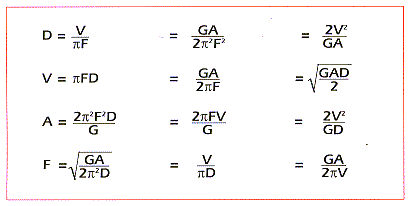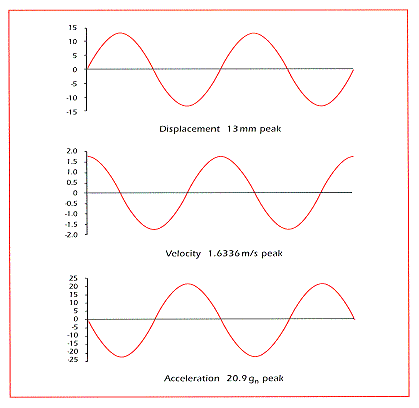Perform Multi-Dimensional Displacement Sensing Within 10 Minutes

Air Data Products

3D Displacement Sensors

Special Offers

Support

Sinusoidal Motion Calculator
 2. Select the column for the variables you know. -> 1. Select a row below (D, V, A, or F). 4. Enter the variables you know below. D Displacement (peak-to-peak) V = F = A = F = V = A = V Velocity (peak) F = D = A = F = A = D = A Acceleration (peak) F = D = F = V = V = D = F Frequency A = D = V = D = A = V = 3. Select your measurement system below. Units D = V = A = F = Metric mm mm/s gn Hz Imperial in in/s gn Hz SI mm mm/s m/s² Hz 5. Press Calculate Answer For the above values, the frequency (F) result is 5.30516.

To use the above calculator:

1. Select what variable you want to solve for by selecting the appropriate radio button in the first column (D, V, A, or F).
2. Select what set of variables you want to use for the calculation by selecting the appropriate radio button in the top row (select the radio button that corresponds to the variables you know).
3. Select the measurement system of your choice in the last row (Metric, Imperial, or SI).
4. Enter the values of the variables in the table cell that is the intersection of your choices in items 1 and 2 above. Use the units of measure shown for the measurement system of your choice.
5. Press "Calculate" for the answer.

There is a mathematical relationship between frequency, displacement, velocity, and acceleration for sinusoidal motion when considering their peak values. The relationship is such that if any two of the four variables are known, the other two can be calculated. The equations below provide all the combinations required.Equations for Sinusoidal Motion
Displacement (D), Velocity (V), Acceleration (A), and Frequency (F)

G in these formulas is not the acceleration of gravity. It is a constant for calculation within different systems. For metric, G is 9.80665 m/s². For Imperial, G is 386.0885827 in/s² For SI, G is 1 m/s²

Since the motion is sinusoidal, the displacement, velocity, and acceleration are changing sinusoidally. However, they are not in phase. The phase relationship between displacement, velocity, and acceleration is that such that velocity is 90° out of phase with acceleration and displacement is 180° out of phase with acceleration.20 Hz Sinusoidal Motion

Another use of this calculator is to determine the maximum frequency responses for SpaceAge Control position transducers. To do this, refer to data sheets located in our (Literature Room) and note the maximum acceleration for a given model. Then, use this calculator to determine the model's maximum frequency for a given displacement and related information.

Equations, graphs, and information are courtesy of Richard Baker who produces VIBKIT, a comprehensive vibration testing toolkit that has a demo version available.

Note: 1 gn = 9.80665 m/s² = 32.174 ft/s² = 386.0886 in/s².

Other calculators:

No Warranties: This calculator and information are provided "as is" without any warranty, condition, or representation of any kind, either express or implied, including but not limited to, any warranty respecting non-infringement, and the implied warranties of conditions of merchantability and fitness for a particular purpose. In no event shall SpaceAge Control, Inc. be liable for any direct, indirect, special, incidental, consequential or other damages howsoever caused whether arising in contract, tort, or otherwise, arising out of or in connection with the use or performance of the information contained on this Web page.